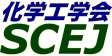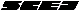## \$B9V1i%W%m%0%i%`!J2q>l!&F|DxJL!K(B

### U\$B2q>l(B

\$B:G=*99?7F|;~!'(B2012-08-20 15:02:17

\$BBh(B1\$BF|(B
\$B9V1i(B
\$B;~9o(B
\$B9V1i(B
\$BHV9f(B
\$B9V1iBjL\!?H/I=\$B%-!<%o!<%I(B\$BJ,N`(B
\$BHV9f(B
\$B\$BHV9f(B
\$B%7%s%]%8%&%`(B \$B!c@:L)9bEY2=!&B?MM2=\$9\$k%_%-%7%s%05;=Q!d(B
(10:00\$B!A(B11:00)\$B!!(B(\$B:BD9(B \$B>1Ln(B \$B8|(B)
10:00\$B!A(B 10:20U104Kenics Static Mixer\$B\$rMQ\$\$\$?J*@-\$N0[\$J\$kN.BN\$N:.9gFC@-(B
(\$B7DXfBgM}9)(B) \$B!{(B(\$B3X(B)\$BKv8w(B \$B@5E5(B \$B!&(B (\$B3X(B)\$B5WJ](B \$B5.B'(B \$B!&(B (\$B6&(B)\$B2#?9(B \$B9d(B \$B!&(B (\$B@5(B)\$B?"ED(B \$BMx5W(B
Kenics Static Mixer
mixing
properties
S-2670
10:20\$B!A(B 10:40U105\$B9bG4@-N.BNCf\$K\$*\$1\$kN3;RIbM7(B
(\$B;37ABg1!M}9)(B) \$B!{(B(\$B3X(B)\$BK\4V(B \$BMN(B \$B!&(B (\$B;37ABg(B) (\$B@5(B)\$B9b66(B \$B9,;J(B \$B!&(B (\$B@5(B)\$B9bH*(B \$BJ]G7(B
Suspension of particles
high viscosity fluid
non-newtonian fluids
S-26786
10:40\$B!A(B 11:00U106\$BG[4I<4J}8~\$NG;EY%`%i\$rDc8:\$9\$k@E;_7?%_%-%5\$K\$*\$1\$k(BCFD\$B\$rMQ\$\$\$?:.9g@-G=:GE,2=(B
(\$B00M-5!:`9)6H(B) \$B2VED(B \$BIR9-(B \$B!&(B \$B!{2,ED(B \$B5.90(B \$B!&(B \$B9uED(B \$B7r;J(B \$B!&(B \$B>>2<(B \$B9,B@O:(B \$B!&(B (\$B;37ABg(B) (\$B@5(B)\$B9b66(B \$B9,;J(B
In-line mixing
CFD
Time difference
S-26871
(11:00\$B!A(B12:00)\$B!!(B(\$B:BD9(B \$B?N;V(B \$BOBI'(B)
11:00\$B!A(B 11:20U107(\$B9V1iCf;_(B)

100443
11:20\$B!A(B 11:40U108\$B5\$1U<+M33&LL>e\$KH/@8\$9\$kI=LL8z2L\$N%^%\$%/%m:.9gB%?J\$X\$N1~MQ(B
(\$B.Ln(B \$BD> \$B!&(B (\$B
Micromixing
Gas-liquid free interface
Marangoni effect
S-26606
11:40\$B!A(B 12:00U109\$B%^%\$%/%m%_%-%5!<\$N:.9g!&EAG.FC@-I>2A\$X\$N2=3XH?1~\$NE,MQ(B
(\$BElM}Bg9)(B) \$B!{(B(\$B@5(B)\$B>.NS(B \$BBgM4(B \$B!&(B (\$BElM}Bg1!Am2=(B) (\$B3X(B)\$B@P0f(B \$BM' \$B!&(B (\$BElM}Bg9)(B) (\$B@5(B)\$B9b66(B \$BCR51(B \$B!&(B (\$B@5(B)\$BBgC](B \$B>!?M(B \$B!&(B (\$B@5(B)\$B>1Ln(B \$B8|(B \$B!&(B (\$BF|L}(B) (\$B@5(B)\$BB@ED(B \$B=SI'(B
Micro mixer
Mixing performance
Heat transfer performance
S-26531
(13:00\$B!A(B13:40)\$B!!(B(\$B;J2q(B \$B5H@n(B \$B;KO:(B)
13:00\$B!A(B 13:40U113[\$BE8K>9V1i(B]\$B%_%-%7%s%0AuCV\$K\$*\$1\$k?tCMN.F02r@O(B(CFD)\$B\$HEE5\$Dq93J}<0%H%b%0%i%U%#!<(B(ERT)\$B\$NE,MQ\$H\$=\$NE83+(B
(\$B2#9qBg1!9)(B) \$B!{(B(\$B@5(B)\$B>e%N;3(B \$B<~(B
Mixing Equipment
Computational Fluid Dynamics
Electrical Resistance Tomography
S-26152
(13:40\$B!A(B14:40)\$B!!(B(\$B:BD9(B \$B?"ED(B \$BMx5W(B)
13:40\$B!A(B 14:00U115CFD\$B\$rMQ\$\$\$?JQ7A%G%#%9%/%?!<%S%sMc\$NFC@-M=B,(B
(\$BJ!2,Bg9)(B) \$B!{(B(\$B3X(B)\$BD+0f(B \$BM:0lO/(B \$B!&(B (\$B@5(B)\$BNk@n(B \$B0l8J(B
CFD
Power number
Fractal impeller
S-26384
14:00\$B!A(B 14:20U116\$B?tCMN.F02r@O\$rMQ\$\$\$?8G1U3IYBAeFb\$K\$*\$1\$k3IYB1):,\$X\$NN3;R>WFM8=>]\$NDjNL2=\$N8!F\$(B
(\$B2#9qBg9)(B) \$B!{(B(\$B3X(B)\$B2CF#(B \$BM&?M(B \$B!&(B (\$B@5(B)\$B;03Q(B \$BN4B@(B \$B!&(B (\$B@5(B)\$B?N;V(B \$BOBI'(B \$B!&(B (\$B@5(B)\$B>e%N;3(B \$B<~(B
CFD
solid-liquid mixing
particle collision
S-26195
14:20\$B!A(B 14:40U117\$B2sE>1_E{7?1U1UCj=PAuCV\$K\$*\$1\$kN.F0>uBV\$K5Z\$\\$9AuCV%9%1!<%k\$N1F6A\$N8!F\$(B
(\$B2#9qBg9)(B) \$B!{(B(\$B3X(B)\$B9l(B \$B7E(B \$B!&(B (\$B@5(B)\$B;03Q(B \$BN4B@(B \$B!&(B (\$B@5(B)\$B?N;V(B \$BOBI'(B \$B!&(B (\$B@5(B)\$B>e%N;3(B \$B<~(B \$B!&(B (\$B86;RNO5!9=(B) \$BJ?Ln(B \$B909/(B \$B!&(B \$B2.Ln(B \$B1Q \$B!&(B \$B:4Ln(B \$BM:0l(B \$B!&(B \$B:dK\(B \$B=_;V(B
mixing
liquid-liquid extraction
scale effect
S-26201
(14:40\$B!A(B15:40)\$B!!(B(\$B:BD9(B \$B>.NS(B \$BBgM4(B)
14:40\$B!A(B 15:00U118\$BCf9bG4EY1U\$rBP>]\$H\$7\$?%^%C%/%9%V%l%s%IMcJP?43IYB\$K\$*\$1\$kN.F0>uBV\$H:.9g@-G=(B
(\$B2#9qBg9)(B) \$B!{(B(\$B@5(B)\$B?N;V(B \$BOBI'(B \$B!&(B (\$B@5(B)\$B;03Q(B \$BN4B@(B \$B!&(B (\$B@5(B)\$B>e%N;3(B \$B<~(B
eccentric mixing
maxblend
mixing performance
S-26318
15:00\$B!A(B 15:20U119\$B%/%j!<%`Nd5QJ];}Ae\$K\$*\$1\$k3IYBMc7A>u\$,Nd5QB.EY\$K5Z\$\\$91F6A(B
(\$B?91JF}6H(B/\$B2#9qBg1!9)(B) \$B!{(B(\$B@5(B)\$B5\K\(B \$BE/IW(B \$B!&(B (\$B?91JF}6H(B) (\$B@5(B)\$B@_3Z(B \$B1QIW(B \$B!&(B (\$B2#9qBg1!9)(B) (\$B@5(B)\$B?N;V(B \$BOBI'(B \$B!&(B (\$B@5(B)\$B;03Q(B \$BN4B@(B \$B!&(B (\$B@5(B)\$B>e%N;3(B \$B<~(B
agitation
cooling
cream
S-26124
15:20\$B!A(B 15:40U120\$B
(\$B%]%j%W%i(B) \$B!{(B(\$B@5(B)\$BJ?ED(B \$BK.9I(B \$B!&(B \$B@PED(B \$BBg(B \$B!&(B \$BJ?74(B \$B850l(B \$B!&(B (\$B6eBg9)(B) (\$B@5(B)\$BL>2E;3(B \$B>MLi(B \$B!&(B (\$B@5(B)\$B3a86(B \$BL->0(B
Twin-Screw Extruder
Simulation
Polymer Compounding
S-2637

\$BBh(B2\$BF|(B
\$B9V1i(B
\$B;~9o(B
\$B9V1i(B
\$BHV9f(B
\$B9V1iBjL\!?H/I=\$B%-!<%o!<%I(B\$BJ,N`(B
\$BHV9f(B
\$B\$BHV9f(B
\$B%7%s%]%8%&%`(B \$B!c@:L)9bEY2=!&B?MM2=\$9\$k%_%-%7%s%05;=Q!d(B
(9:00\$B!A(B10:00)\$B!!(B(\$B:BD9(B \$BKY9>(B \$B9';K(B)
9:00\$B!A(B 9:20U201\$BB.EYJQF0Aj4X\$K4p\$E\$\$\$?YxYBAeEG=PN.0h\$N12\$K4X\$9\$k9M;!(B
(\$BEl9)Bg1!M}9)(B) \$B!{(B(\$B3X(B)\$B0pMU(B \$B=TM5(B \$B!&(B (\$B@5(B)\$B5H@n(B \$B;KO:(B \$B!&(B (\$B@5(B)\$BBg@n86(B \$B??0l(B
Eddy
Discharge Flow
Velocity Fluctuation
S-26555
9:20\$B!A(B 9:40U202\$B5!3#E*2sE>McYxYBAe\$K\$*\$1\$kMc2sE>NN0hFb\$N1UN.\$l(B
(\$B?73cBg9)(B) \$B!{(B(\$B3X(B)\$BEgED(B \$BD> \$B!&(B (\$B3X(B)\$BG_DE(B \$B7r4u(B \$B!&(B (\$B@5(B)\$B5HED(B \$B2mE5(B
mixing
impeller region
fluid flow
S-26616
9:40\$B!A(B 10:00U203\$B5!3#E*2sE>McYxYBAe7?H?1~4o\$K\$*\$1\$k1UAj:.9g(B
(\$B?73cBg9)(B) \$B!{(B(\$B3X(B)\$B?{Ln(B \$BNh(B \$B!&(B (\$B3X(B)\$BG_DE(B \$B7r4u(B \$B!&(B (\$B@5(B)\$B5HED(B \$B2mE5(B
mixing
reactor
selectivity
S-26618
(10:00\$B!A(B11:00)\$B!!(B(\$B:BD9(B \$B2CF#(B \$BDw?M(B)
10:00\$B!A(B 10:20U204\$B@55U8r8_2sE>Mc\$N?6F0>r7o\$,AeFbN.F0>uBV\$K5Z\$\\$91F6A(B
(\$B?@8MBg1!9)(B) \$B!{(B(\$B3X(B)\$B@gED(B \$BAa5*(B \$B!&(B (\$B?@8MBg9)(B) \$B;3>e(B \$BE5G7(B \$B!&(B (\$B?@8MBg1!9)(B) (\$B@5(B)\$B8VED(B \$B1YG7(B \$B!&(B (\$B%"!<%k%U%m!<(B) (\$B@5(B)\$BC]ED(B \$B9((B \$B!&(B (\$B:eBg(B) (\$B@5(B)\$BJ?ED(B \$BM:;V(B \$B!&(B (\$B?@8MBg9)(B) (\$B@5(B)\$BF|=P4V(B \$B\$k\$j(B \$B!&(B (\$B?@8MBg1!9)(B) (\$B@5(B)\$BNkLZ(B \$BMN(B
vortex
flow condition
S-26880
10:20\$B!A(B 10:40U205\$B29EYJQF0>l\$K\$*\$1\$k3IYBAeFb\$N8IN):.9gNN0h\$NF0E*5sF0(B
(\$B?@8MBg1!9)(B) \$B!{(B(\$B3X(B)\$BEDCf(B \$B5WEP(B \$B!&(B (\$B@5(B)\$B7'C+(B \$B595W(B \$B!&(B (\$B@5(B)\$BKY9>(B \$B9';K(B \$B!&(B (\$B@5(B)\$BBgB<(B \$BD>?M(B
Stirred vessel
Isolated mixing region
Laminar mixing
S-26209
10:40\$B!A(B 11:00U206\$B8G(B-\$B1U7O3IYB\$K\$*\$1\$kO"B3H4\$-=P\$7A`:n\$KE,\$7\$?3IYBJ}K!!&Mc\$K\$D\$\$\$F(B
(\$B:4C]2=3X5!3#9)6H(B) \$B!{:4!9LZ(B \$B7r2p(B \$B!&(B \$B6b?9(B \$B5W9,(B \$B!&(B (\$B@5(B)\$B2CF#(B \$B9%0l(B
Mixing
Solid-Liquid
Distribution
S-26202
(11:00\$B!A(B12:00)\$B!!(B(\$B:BD9(B \$B;03Q(B \$BN4B@(B)
11:00\$B!A(B 11:20U207\$B3IYB=jMWF0NO\$K5Z\$\\$9FbAuJ*\$N8z2L(B
(\$BL>9)Bg(B) \$B!{(B(\$B3X(B)\$B8E@n(B \$BM[51(B \$B!&(B (\$B@5(B)\$B2CF#(B \$BDw?M(B \$B!&(B (\$B@5(B)\$BB?ED(B \$BK-(B
Mixing
Draft tube
Coil
S-26243
11:20\$B!A(B 11:40U208\$BBg7?3IYBMc\$NF0NO@~?^\$H:.9g%Q%?!<%s\$N4X78(B
(\$BL>9)Bg(B) (\$B@5(B)\$B2CF#(B \$BDw?M(B \$B!&(B \$B!{(B(\$B@5(B)\$B2CF#(B \$BCNHA(B \$B!&(B (\$B@5(B)\$BB?ED(B \$BK-(B
mixing
power consumption
impeller
S-26265
11:40\$B!A(B 12:00U209[\$BM%=(O@J8>^(B]\$BA/L@\$JN.L.@~\$N2D;k2=K!(B
(\$B:eBg1!4p9)(B) (\$B@5(B)\$B66K\(B \$B=SJe(B \$B!&(B \$B@>@n(B \$BC#:H(B \$B!&(B \$B?7Ln(B \$B=c0l(B \$B!&(B \$B!{(B(\$B3X(B)\$B@>B<(B \$BN<=S(B \$B!&(B (\$B@5(B)\$B0f>e(B \$B5AO/(B
Visualization
Streakline
Surfactant
S-2614

\$B9V1i%W%m%0%i%`(B
\$B2=3X9)3X2q(B \$BBh(B44\$B2s=)5(Bg2q(B(C) 2012 \$B8x1W
Most recent update: 2012-08-20 15:02:17
For more information contact \$B2=3X9)3X2qElKL;YIt(B \$BBh(B44\$B2s=)5(Bg2q(B \$BLd\$\$9g\$;78(B
E-mail: inquiry-44fwww3.scej.org
This page was generated byeasp 2.28; proghtml 2.28c (C)1999-2011 kawase